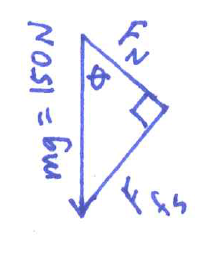## Sabtu, 12 Oktober 2013

### Block on an incline -- trig identity or pythagorean?Joel Houghton from Raleigh writes in about a problem I use in my AP class.  The block shown sits at rest on an incline.  The coefficient of static friction is 0.30.  What is the angle of the incline, and what is the normal force acting on the block?

(As an aside, usually this question is phrased with "maximums":  The MAXIMUM static friction coefficient is 0.30, so what is the BIGGEST angle at which the block can stay at rest?  I assign this problem in week 2 of my AP course, so I ignore the issue of maximums... as I stated it, the problem is still technically correct.)Pythagorean Method:  Joel created a triangle out of force vectors. The 150 N weight acts straight down.  The normal force acts perpendicular to the incline.  The friction force (equal to 0.30 times the normal force) acts up the incline.  Thus, the weight is the hypotenuse of a triangle -- look at the diagram to the right, which Joel sent me.

Joel applied the pythagorean theorem to get an equation in a single variable:

(150 N)2 = Fn2 + (0.30 Fn)2

Joel solved for Fn to get 144 N.  Then he used the cosine function to get an angle of 16 degrees for the incline.

Vector Components and Trig Identity Method:  Joel's method is complete and correct.  I don't personally like to teach students to use a pythagorean approach here, though -- it is very difficult for first-year physics students to see, understand, and be able to create the triangle of force vectors.  Instead, I encourage an identical approach to every problem:  Break angled forces into components, then (for equilibrium) set up=down, left=right.

The weight breaks up into components down the plane (mgsinθ) and perpendicular to the plane (mgcosθ).  The two equilibrium equations become:

(0.30)Fn = mgsinθ
Fn = mgcosθ

Solving for Fn requires the trig identity that sin/cos = tan.  I tell my class that this is the ONLY trig identity they will be expected to use in AP physics... but this identity is in fact used relatively frequently.  Here, dividing the equations by each other yields tan θ = 0.30... which means, the angle of the plane is 16 degrees.

You solve this problem your way.  I merely pose two possible approaches.